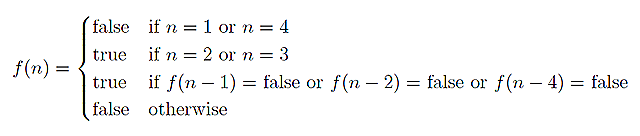# 一道面试题（Nim取子游戏）——如何将数学思维应用到编程中

## 寻找思路

n 颗石子，两个人轮流取子，每次只能取一颗、两颗或者四颗石子，最后取完石子的人为负。问，先取子的人还是后取子的人有必胜策略。

n = 1 时，先取子的人必然取完全部的石子，因此，先取子的人必负，从而后取子的人必胜；即，当 n = 1 时，后取子的人有必胜策略。

n = 2 时，先取子的人可以只取一颗石子，从而后取子的人进入上述 n = 1 时先取子的情况；因此，当 n = 2 时，先取子的人有必胜策略。

n = 3 时，先取子的人可以只取两颗石子，从而后取子的人进入上述 n = 1 时先取子的情况；因此，当 n = 3 时，先取子的人有必胜策略。

n = 4 时，先取子的人若只取一颗石子，则后取子的人将进入上述 n = 3 时先取子的情况，后取子的人必胜；若先取子的人只取两颗石子，则后取子的人将进入上述 n = 2 时先取子的情况，后取子的人必胜；先取子的人若取四颗石子，则恰将所有石子取光，从而后取子的人必胜；综上所述，当 n = 4 时，后取子的人有必胜策略。

n = 5 时，先取子的人若只取一颗石子，则后取子的人将进入上述 n = 4 时先取子的情况，从而必输；因此，当 n = 5 时，先取子的人有必胜策略。

* P N N P N N P N N P  ……

0 1 2 3 4 5 6 7 8 9 10 ……

## 形式化的证明

n mod 3 = 1 时，游戏处于P状态；其余情况，游戏处于N状态。

n = 1 时，游戏处于P状态；当 n = 2 时，游戏处于N状态；当 n = 3 时，游戏处于N状态。

n mod 3 = 0，则：(n – 3)处于N状态，(n – 2)处于P状态，(n – 1)处于N状态，n 处于N状态；

n mod 3 = 1，则：(n – 3)处于P状态，(n – 2)处于N状态，(n – 1)处于N状态，n 处于P状态；

n mod 3 = 2，则：(n – 3)处于N状态，(n – 2)处于N状态，(n – 1)处于P状态，n 处于N状态。

n mod 3 = 0，则由于从状态(n + 1)仅可以转换到状态 n、状态(n - 1)、状态(n - 3)，而这三个状态均为N状态，因此状态(n + 1)为P状态；（n + 1 mod 3 = 1）

n mod 3 = 1，则由于从状态(n + 1)可以转换到状态 n，而状态 n 为P状态，因此状态(n + 1)为N状态；（n + 1 mod 3 = 2）

n mod 3 = 2，则由于从状态(n + 1)可以转换到状态(n - 1)，而状态(n - 1)为P状态，因此状态(n + 1)为N状态。（n + 1 mod 3 = 0）

## 递归形式bool f(int n)
{
if(n == 1 || n == 4) return false;
if(n == 2 || n == 3) return true;
if( !f(n - 1) || !f(n - 2) || !f(n - 4) ) return true;
return false;
}

## 动态规划解

bool f(int n)
{
if(n <= 0) return false;
bool *states = (bool *)malloc(sizeof(bool) * n);
states = states = false;
states = states = true;
for(int i = 4; i < n; i++) {
states[i] = !(states[i - 1]
&& states[i - 2]
&& states[i - 4]);
/* According to De Morgan's laws */
}
return states[n - 1];
}

bool fn(int n)
{
if(n <= 0) return false; /* We can return anything, because it has no real meaning. */
if(n == 1 || n == 4) return false;
if(n == 2 || n == 3) return true;
/* a ~ fn(i - 4)
* b ~ fn(i - 3)
* c ~ fn(i - 2)
* d ~ fn(i - 1)
* f ~ fn(i)
*/
bool a = d = false, b = c = true;
for(int i = 5; i <= n; i++) {
bool f = !(a && c && d);
a = b; b = c; c = d; d = f;
}
return d;
}

## 进阶话题

《编程之美》P67~87

Sprague-Grundy Function-SG函数--博弈论(3)

posted @ 2012-04-24 17:12  HCOONa  阅读(2610)  评论(0编辑  收藏  举报